# Solutions

As mentioned within the session setup, load the following packages using the `library()` function. Additionally, as we will be using a data set with large numbers, set scipen to 999 using the option function.

``````  library(tidyverse)
library(RColorBrewer)

options(scipen = 999)``````

Furthermore, for the purpose of this session, we will be using data from the World Bank Open Data. In particular we will be using a collection of variables from 1999, these variables were selected to provide us plenty of room to explore!

``WDB_1999 <- read_csv("../data/WDB_1999.csv")``

Section 1: ggplot2 vs plot

Exercise 1: Plotting birthrate against deathrate using both the `plot()` and `ggplot()` function, discuss which has more potential in displaying data clearly.

``````## Plotting using plot()

plot(data = WDB_1999,
birthrate ~ deathrate)````````````## Plotting using ggplot()

ggplot(data = WDB_1999,
mapping = aes(
x = deathrate,
y = birthrate
)) +
geom_point()``````
``## Warning: Removed 17 rows containing missing values (geom_point).``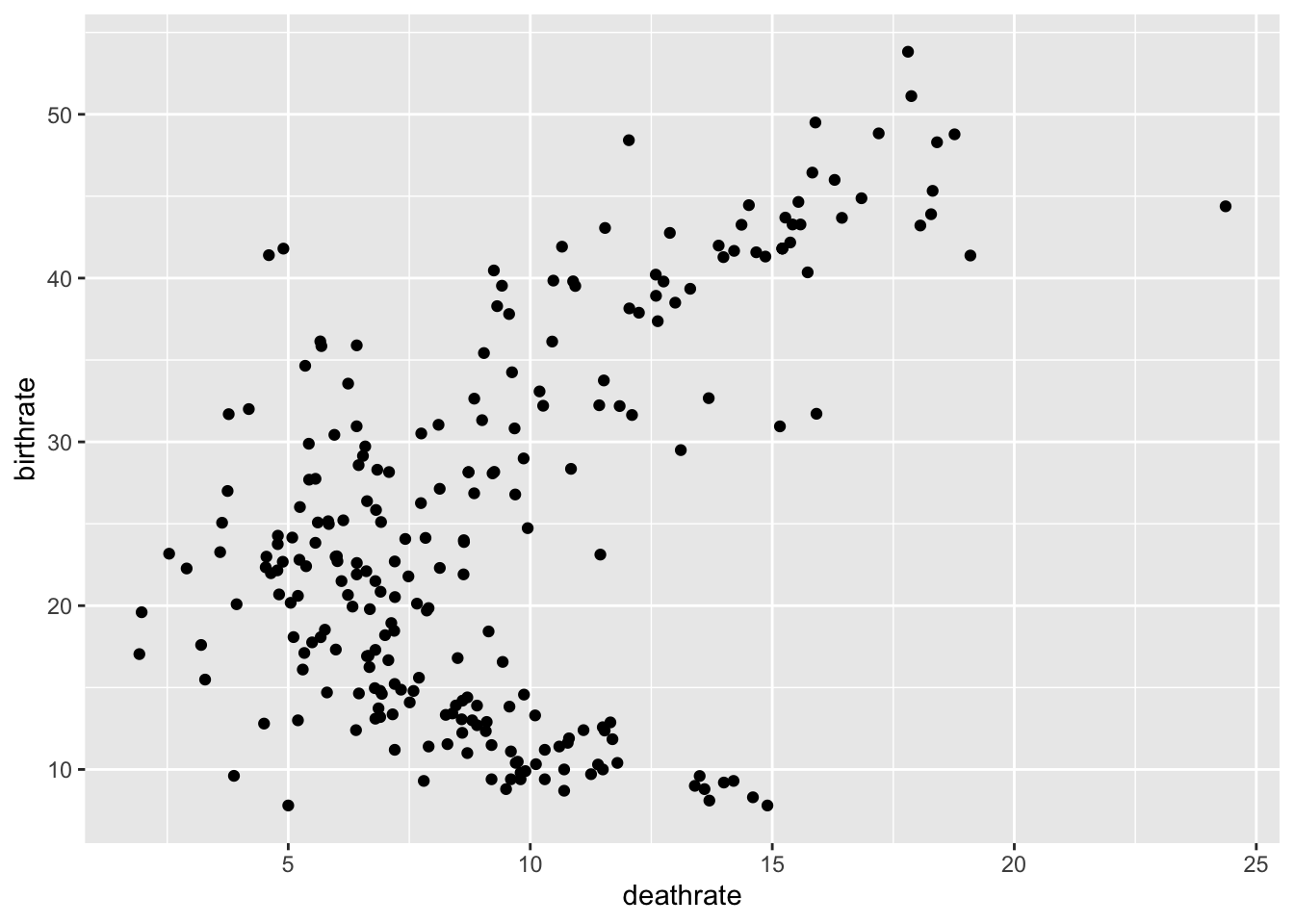Exercise 2: Expand the plot to group these points by Continent, which provides us with more information and is easier to achieve? Remember, you’ll need to recode WDB_1999\$Continent as a factor using the function:

``````## Plotting using plot()

WDB_1999\$Continent <- as.factor(WDB_1999\$Continent)

plot(data = WDB_1999,
birthrate ~ deathrate,
col = c("blue", "light blue", "red", "pink",
"green", "dark green", "black", "purple")[Continent])``````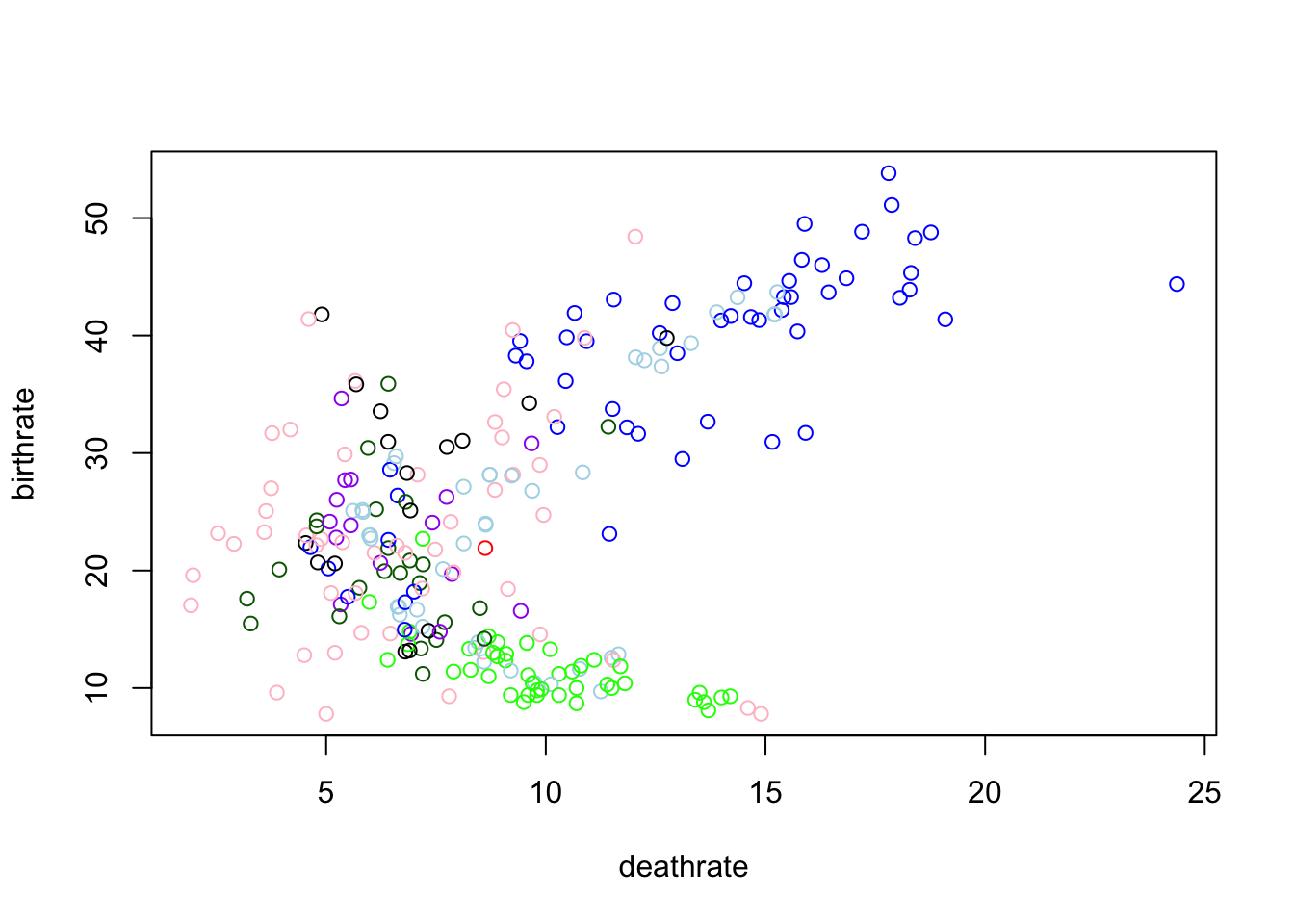``````## Plotting using ggplot()

ggplot(data = WDB_1999,
mapping = aes(
x = deathrate,
y = birthrate,
colour = Continent
)) +
geom_point()``````
``## Warning: Removed 17 rows containing missing values (geom_point).``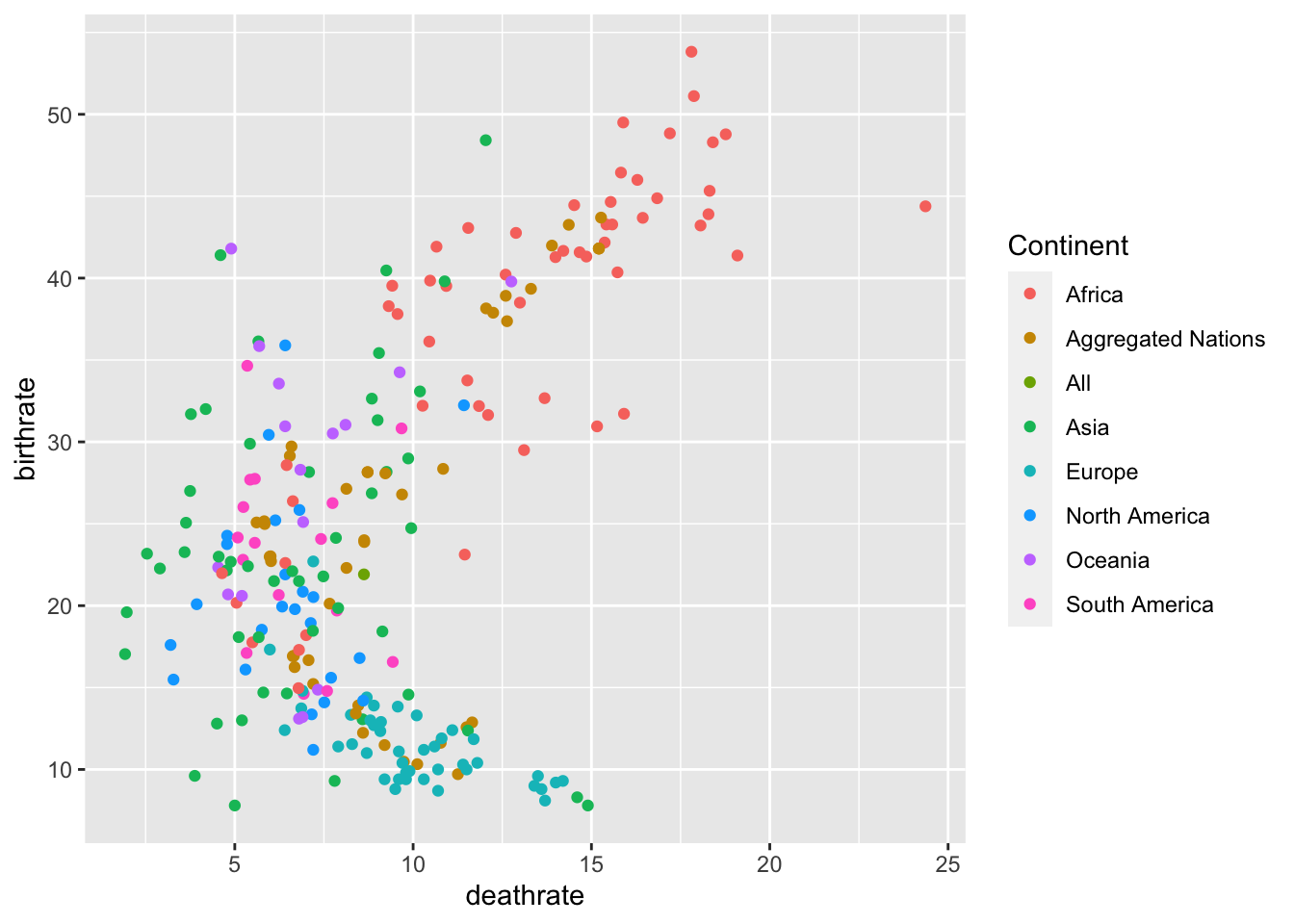Section 2: Scatter Plots in ggplot

Exercise 3: Change the size parameter to ed.years to see if there is a trend between amount of years in Education and the Birth and Death Rate, set the alpha parameter to 0.5 to clearly see the relationships.

``````  ggplot(data = WDB_1999,
mapping = aes(
x = birthrate,
y = deathrate,
colour = Continent,
size = ed.years
)) +
geom_point(alpha = 0.5)``````
``## Warning: Removed 69 rows containing missing values (geom_point).``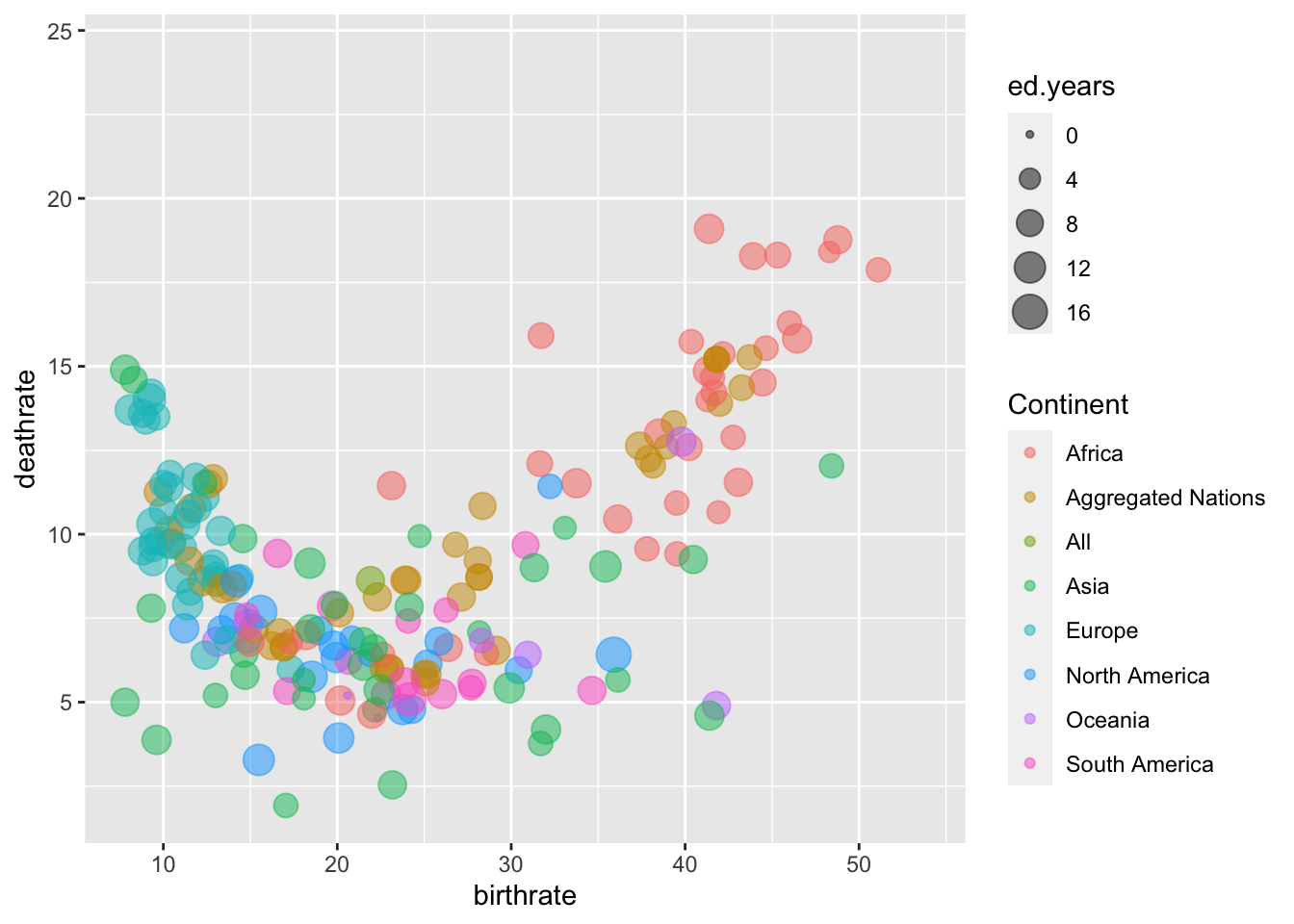Exercise 4: Change the Labels on the X and Y axis’ and provide a suitable title for the graph

``````  ggplot(data = WDB_1999,
mapping = aes(
x = birthrate,
y = deathrate,
colour = Continent,
size = ed.years
)) +
geom_point(alpha = 0.5) +
labs(x = "Birth Rate",
y = "Death Rate",
title = "Interaction between Birth and Death Rate.")``````
``## Warning: Removed 69 rows containing missing values (geom_point).``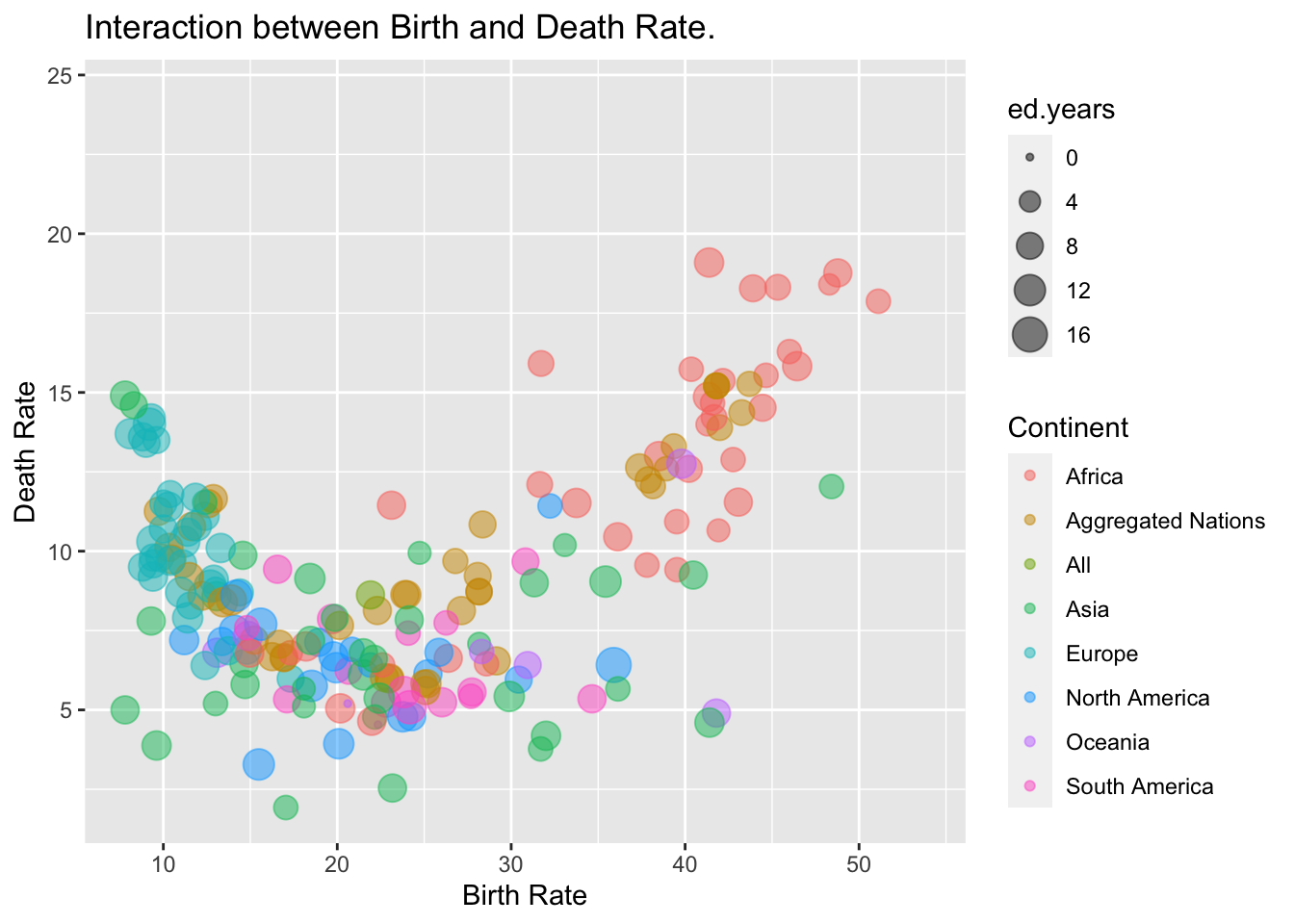Section 3: Bar Charts and Histograms

Exercise 5: Using the parameter `stat = "identity"` within the `geom_bar()` function, create a bar chart of `Continent` plotted against the mean `birthrate` or `deathrate`

``````  ggplot(data = WDB_1999) +
geom_bar(stat = "identity",
mapping = aes(x = Continent,
y = mean(birthrate, na.rm = TRUE))) ``````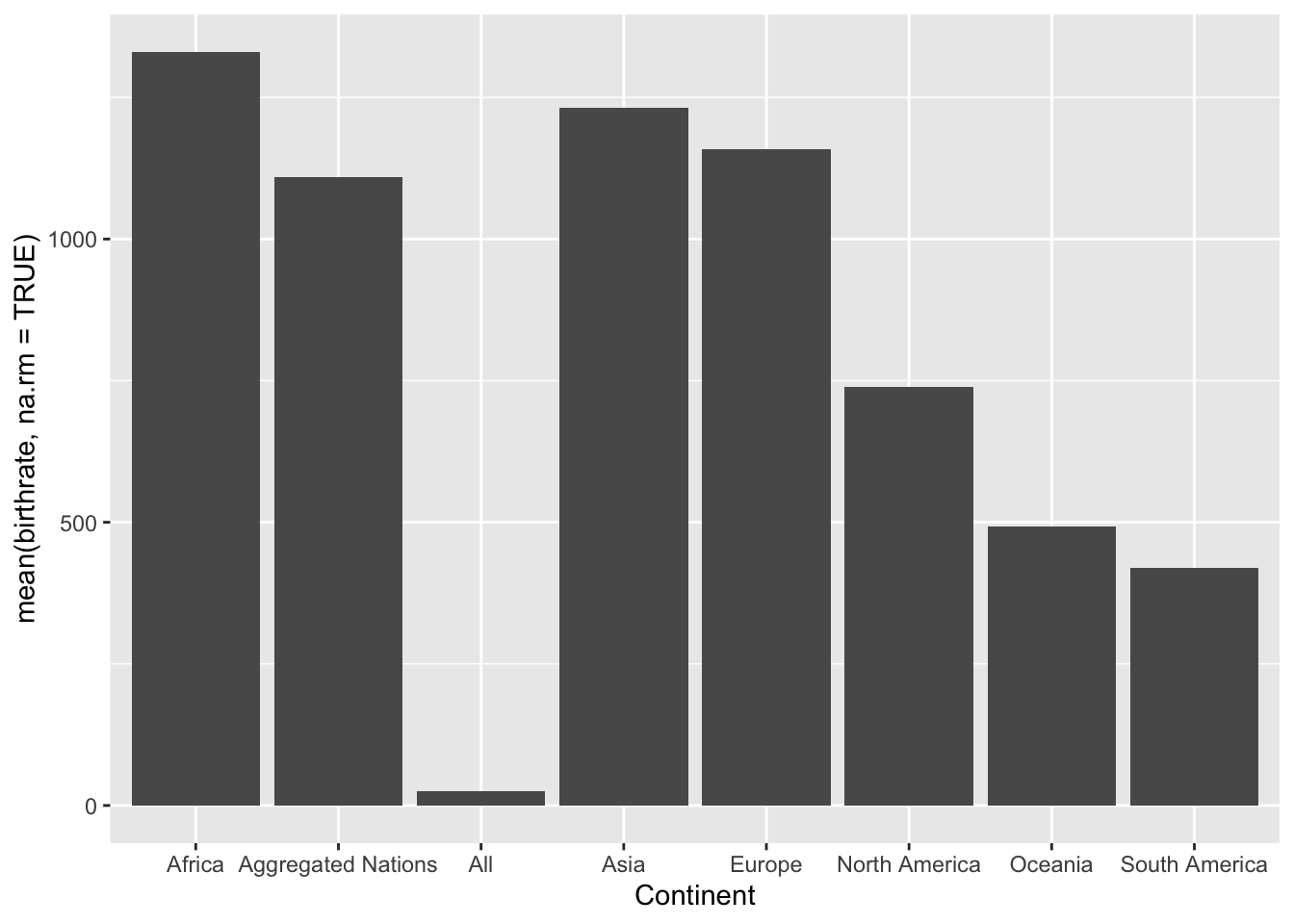``````  ggplot(data = WDB_1999) +
geom_bar(stat = "identity",
mapping = aes(x = Continent,
y = mean(deathrate, na.rm = TRUE))) ``````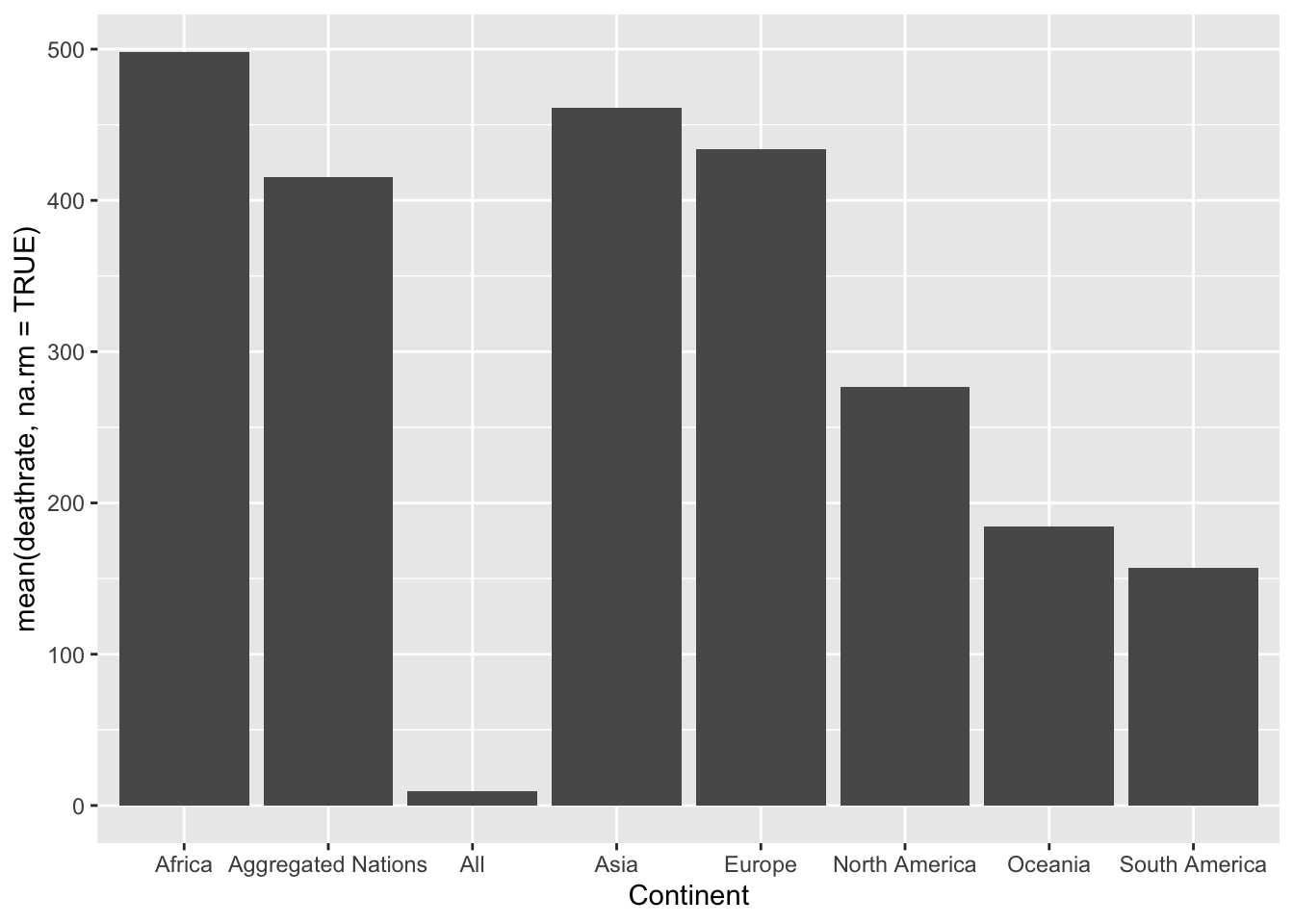Exercise 6: Using the function `geom_histogram()` create a histogram of the birthrate and deathrate

``````  ggplot(data = WDB_1999) +
geom_histogram(mapping = aes(x = birthrate), colour = "blue", alpha = 0.5) +
geom_histogram(mapping = aes(x = deathrate), colour = "red", alpha = 0.5)``````
``## `stat_bin()` using `bins = 30`. Pick better value with `binwidth`.``
``## Warning: Removed 16 rows containing non-finite values (stat_bin).``
``## `stat_bin()` using `bins = 30`. Pick better value with `binwidth`.``
``## Warning: Removed 17 rows containing non-finite values (stat_bin).``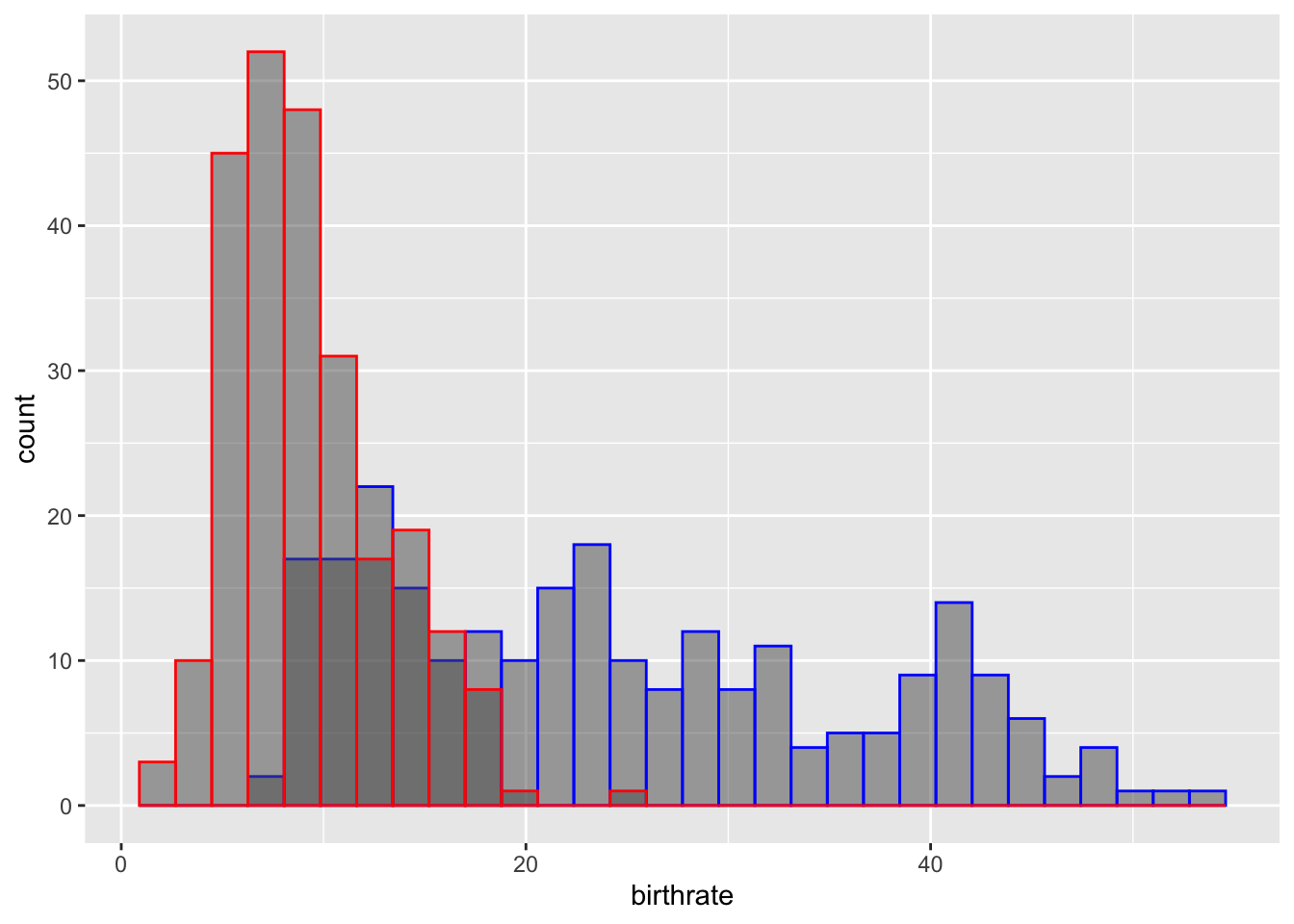Section 4: Adding density plots to Histograms

Exercise 7: Using the plot created in exercise 6, add the y-variable `..density..` and `binwidth = 1` to `geom_histogram()` in addition to adding `geom_density()` to add density lines to the Histogram

``````  ggplot(data = WDB_1999) +
geom_histogram(mapping = aes(x = birthrate, y = ..density..), binwidth = 1,
colour = "blue", alpha = 0.5) +
geom_histogram(mapping = aes(x = deathrate, y = ..density..), binwidth = 1,
colour = "red", alpha = 0.5) +
geom_density(mapping = aes(x = birthrate), colour = "blue", alpha = 0.5) +
geom_density(mapping = aes(x = deathrate), colour = "red", alpha = 0.5)``````
``## Warning: Removed 16 rows containing non-finite values (stat_bin).``
``## Warning: Removed 17 rows containing non-finite values (stat_bin).``
``## Warning: Removed 16 rows containing non-finite values (stat_density).``
``## Warning: Removed 17 rows containing non-finite values (stat_density).``Exercise 8: Add the parameter, `adjust = 2` in the density plot, to smooth this link and make it more easily interpretable

``````  ggplot(data = WDB_1999) +
geom_histogram(mapping = aes(x = birthrate, y = ..density..), binwidth = 1,
colour = "blue", alpha = 0.5) +
geom_histogram(mapping = aes(x = deathrate, y = ..density..), binwidth = 1,
colour = "red", alpha = 0.5) +
geom_density(mapping = aes(x = birthrate), colour = "blue", alpha = 0.5, adjust = 2) +
geom_density(mapping = aes(x = deathrate), colour = "red", alpha = 0.5, adjust = 2)``````

Section 5: Extra Useful Tips and Functions

Exercise 9: Use the `ggsave()` function to save your last plot

``````ggsave(filename = ??,
plot = last_plot())``````